# Eigen values of integral operators, numerical methods

Numerical methods for computing the complete spectrum of an integral operator or a part of it (usually one is required to find one or two eigen values of minimal or maximal modulus).

This is often accompanied by the problem of finding numerical approximations of the eigen functions or, more generally, of the root functions, of a given integral operator, corresponding to the desired eigen values. The most important problem is that of finding the eigen values (and eigen functions) of a Fredholm linear integral operator (cf. Fredholm operator).

## Numerical methods for determining the eigen values of Fredholm integral operators.

The eigen value and eigen function problems for a Fredholm integral operator consist of finding the complex numbersfor which there is a non-trivial solution (in a given class of functions) of the integral equation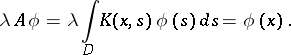(1)

Here,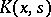is a function (or matrix function) of two groups of variablesandsuch that the integral operator with kernelis a Fredholm operator on the given class of functions, andis a domain in a Euclidean space. The class of functions may be the spaceof continuous functions on, the space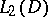of square-integrable functions onor some other function space.

The basic approximation method for solving the eigen value problem (1) is as follows. One chooses some approximation of the integral operator in (1) (see Fredholm equation, numerical methods), e.g., the integral is replaced by a quadrature formula: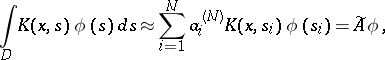(2)

where the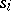are nodes of the quadrature formula and the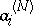are its weights (see ).

Instead of (1), one considers the problem of finding the eigen values and corresponding root spaces of some matrix naturally connected with the approximation (2). Namely,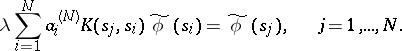(3)

To solve (3), one can use any of the methods of linear algebra for finding eigen values and eigen vectors, or, more generally, root spaces (see Linear algebra, numerical methods in). The resulting eigen values and eigen vectors of the algebraic problem (3) will be close to certain eigen values and eigen vectors of the original problem (1), provided that the operatorsandare close in some specific sense. Instead of (2), other approximations of the integral operator can also be used. The original problem (1) is thus reduced to an algebraic problem analogous to (3). The investigation of the distance between the solutions of the problems (1) and (3) is carried out by methods of functional analysis within the framework of the general theory of approximation methods. The eigen value problem (1) is then treated as a problem of finding the eigen values of some completely-continuous operatoracting on a Banach space:(4)

Problem (3) is treated as the eigen value problem for an operatorwhich is close tobut, generally speaking, acts on another space(related to):(5)

In the general theory of approximation methods, one can prove various theorems about the distance between solutions of problems (4) and (5). As an example of such a statement one can mention the following. Let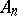be a sequence of operators acting on, and let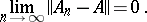Thenwhere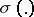is the spectrum of the corresponding operator. In this case, every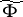coincides with.

The majority of general estimates of the distance between the eigen values and eigen vectors of the approximation problem (5) to those of (4) are not effective: they contain constants whose values are usually not known. To control the precision in such cases one can use a sequence of eigen values (vectors) approximating the desired eigen value (vector) in (1) (or (4)). It is advisable to construct such a sequence without appealing directly to a sequence of problems of the type (5) with successive refinements of, since this course leads to very cumbersome calculations. Instead of this, one can apply various refinement algorithms (for example, based on perturbation theory).

## Generalized eigen value problems.

In applications one also encounters a more general problem than (4), namely that of finding critical parameters of eigen value type. Such problems can be formulated in abstract form as follows.

One has to find values of the parameterfor which the equation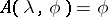(6)

has more than one solution in(whereis some non-linear integral operator on a Banach spacewhich depends on the complex parameter).

In problem (6) there can be further restrictions on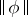and on(for example, one can ask for only thosethat satisfy the condition, whereis given, and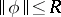).

Problem (6) is closely connected with various problems about bifurcation points in non-linear integral equations. A case of interest is that in whichis linear in, butdoes not enter the equation multiplicatively. The general problem on bifurcation points can be reduced to this form. Moreover, the problem of determining the eigen values of the linear operator (1) that lie in the disc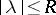, for fixed, reduces to the more general problem (6), in which the operatoris linear inand has a finite-dimensional range. In fact, let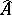be an integral operator with a degenerate kernel and close in norm to:. Equation (1) can be rewritten as: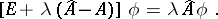If, then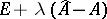is invertible, and the eigen valueswithcan be found from the relation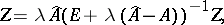(7)

where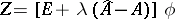. Equation (7) is equivalent (with respect to) to some system of linear algebraic equations. By putting its determinant equal to zero, one obtains an equation whose roots are eigen values of the integral operator (1). This argument is valid in general for an arbitrary completely-continuous operatoron a Banach space, provided that it admits an approximation in norm by operators with a finite-dimensional range. The construction (7) can also be used to obtain a refinement of an approximate eigen value (and eigen function).

The general problem (6) can (by approximating) be reduced by approximation to a finite-dimensional problem of type (6). In the case of more complex problems of this type, the Monte-Carlo method can be used (see ).